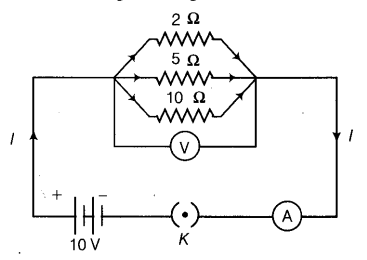# A circuit diagram is given as shown below:

A circuit diagram is given as shown below:Calculate
(i) the current through each resistor.
(ii) the total current in the circuit.
(iii) the total effective resistance of the circuit.